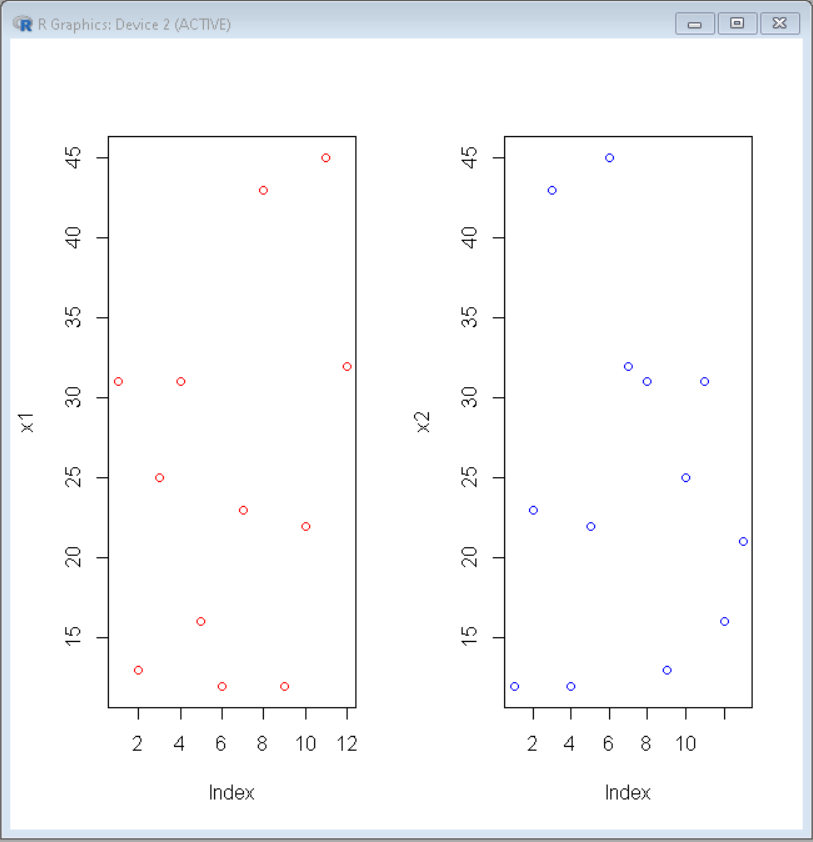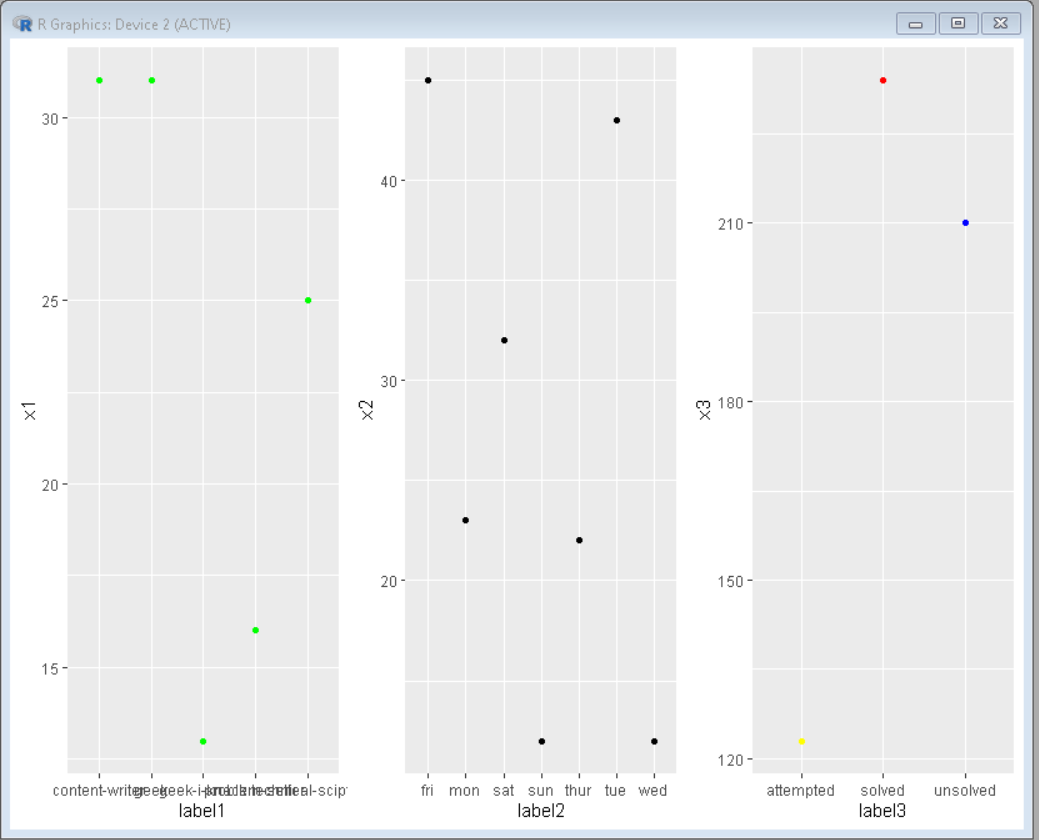# Side by Side Scatter charts in R

• Last Updated : 21 Jul, 2021

In this article, we will discuss how to make side by side scatter plot in R Programming Language.

Method 1: Using par() function

Here we will use par() function to divide frames into grids.

Syntax: par(mfrow, mar, mgp, las)

Parameters:

• mfrow: A numeric vector of length 2, which sets the rows and column in which frame has to be divided.
• mar: A numeric vector of length 4, which sets the margin sizes in the following order: bottom, left, top, and right.
• mgp: A numeric vector of length 3, which sets the axis label locations relative to the edge of the inner plot window.
• las: A numeric value indicating the orientation of the tick mark labels and any other text added to a plot after its initialization.

Here, by changing row and column values, we can draw graphs in them. For drawing side-by-side plots we will keep row as 1 and column as the no. of plots required.

## R

 `# Define data-set columns``x1 <- ``c``(31,13,25,31,16,12,23,43,12,22,45,32)``x2 <- ``c``(12,23,43,12,22,45,32,31,13,25,31,16,21) `` ` `# set the plotting area into a 1*2 array``par``(mfrow = ``c``(1,2))    `` ` `# Draw the two scatter chart using above datasets``plot``(x1, col=``"red"``)``plot``(x2, col=``"blue"``)`

Output:Method 2: Using ggplot2

Here we will use gridExtra contains a function called arrange() that is used to arrange plots as desired.

Syntax: grid.arrange(plot, nrow, ncol)

Parameter:

• plot: ggplot2 plot which we want to arrange
• nrow: Number of rows
• ncol: Number of columns

Here, by changing nrow and ncol values, we can draw graphs in them. For drawing side-by-side plots we will keep nrow as 1 and ncol as the no. of plots required.

## R

 `# Define data-set columns``x1 <- ``c``(31,13,25,31,16)``x2 <- ``c``(12,23,43,12,22,45,32) ``x3 <- ``c``(234,123,210)`` ` `label1 <- ``c``(``'geek'``,``'geek-i-knack'``,``            ``'technical-scipter'``,``            ``'content-writer'``,``            ``'problem-setter'``)`` ` `label2 <- ``c``(``'sun'``,``'mon'``,``'tue'``,``            ``'wed'``,``'thur'``,``'fri'``,``'sat'``)`` ` `label3 <- ``c``(``'solved'``,``'attempted'``,``'unsolved'``)`` ` `# Create data frame using above data column``data1 <- ``data.frame``(x1,label1)``data2 <- ``data.frame``(x2,label2)``data3 <- ``data.frame``(x3,label3)`` ` `# set the plotting area into a 1*3 array``par``(mfrow = ``c``(1,3))`` ` `# import library ggplot2 and gridExtra``library``(ggplot2)``library``(gridExtra)`` ` `# Draw the three scatter chart using above datasets``plot1<-``ggplot``(data1, ``aes``(x=label1, y=x1, group=1)) +``geom_point``(color=``"green"``)`` ` `plot2<-``ggplot``(data2, ``aes``(x=label2, y=x2, group=1)) +``geom_point``()`` ` `plot3<-``ggplot``(data3, ``aes``(x=label3, y=x3, group=1)) +``geom_point``(color=``c``(``"red"``,``"yellow"``,``"blue"``))`` ` `# Use grid.arrange to put plots in columns``grid.arrange``(plot1, plot2, plot3, ncol=3)`

Output:My Personal Notes arrow_drop_up